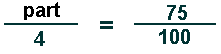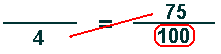# Percentage ProblemsExample 1What number is 75% of 4?   (or   Find 75% of 4.)

The PERCENT always goes over 100.
(It's a part of the whole 100%.)

4 appears with the word of:
It's the WHOLE and goes on the bottom.We're trying to find the missing PART (on the top).

In a proportion the cross-products are equal:   So 4 times 75 is equal to 100 times the PART.

The missing PART equals 4 times 75 divided by 100.

(Multiply the two opposite corners with numbers; then divide by the other number.)

 4 times 75 = 100 times the part 300 = 100 times the part 300/100 = 100/100 times the part 3 = the part